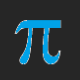# A Euclidean Construction of a Regular Convex Pentagon and a Regular Star Pentagon, Both Inscribed Inside a Given Circle

1. Start with a (green) circle centered on A with radius AB. Point B is on this circle.
2. Construct a line which intersects line AB at point A, in such a way that these two lines are perpendicular.
3. This green circle intersects the newest line at two points. Designate one of these intersections as point C.
4. Bisect segment AC, and mark point D as this segment’s midpoint.
5. Construct a circle (the blue one) which is centered on D and includes point B.
6. The blue circle intersects line AC at two points. Only one of these points is inside the green circle. Label this point F.
7. Construct segment BF. This segment’s length is the edge length of the pentagon under construction.
8. Construct a circle (the red one) which is centered on B and includes point F.
9. The red and green circles have two points of intersection. One of them is closer to point F than the other; label this closer intersection as point I. The other point of intersection is closer to point C; label it J.
10. J, B, and I are vertices of a regular pentagon. Construct a circle (orange) which is centered on point I and passes through point B. The orange and green circles intersect at two points, one of which is labeled B. Label the other one K. Point K is a vertex of the pentagon under construction.
11. Construct a (purple) circle centered on K and passing through I. This purple circle intersects the green circle at two points, one of which is already labeled I. Label the other point of intersection as L. Point L is the fifth vertex of the pentagon.
12. Connect points with segments to form regular pentagon JBIKL.
13. Connect points with segments to form regular star pentagon BKJIL.

## 4 thoughts on “A Euclidean Construction of a Regular Convex Pentagon and a Regular Star Pentagon, Both Inscribed Inside a Given Circle”

1.Anonymous on said:

Hi Robert,

The regular pentagram is constructed together with regular pentagon when you observe at step 6 that the blue circle intersects AC in two points: one inside green circle (point F) and one outside it (let it be E). Then you can observe that BF is the length of pentagon edge (and you observed it) while BE is the length of pentagram edge (you didn’t mentioned this). Next, you can construct the pentagram just like pentagon having its edge length and one of vertices.

Alexandru

Liked by 2 people

2.Paul on said:

This is the third construction of a pentagon inscribed inside a circle that I have learned. Are there others?

Liked by 1 person

•RobertLovesPi on said:

I would guess that there are, but I am not certain.

Like